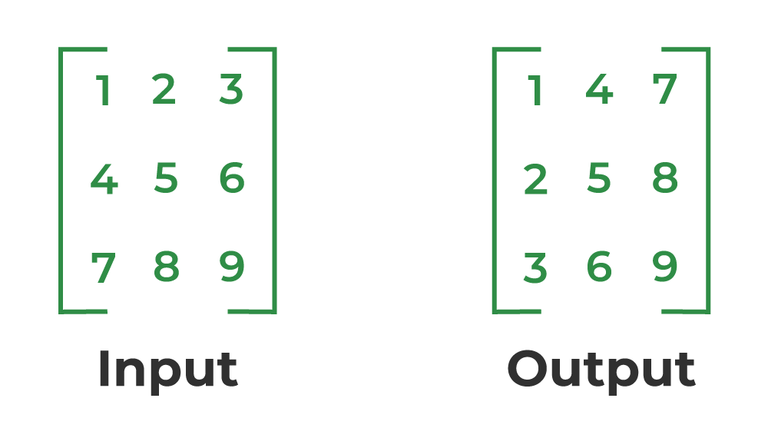# Java Program to Find Transpose of Matrix

Transpose of a matrix is obtained by changing rows to columns and columns to rows. In other words, the transpose of A[][] is obtained by changing A[i][j] to A[j][i].## Find Transpose of a Matrix in Java

There are two shapes in Matrix to find transpose as mentioned below:

• Square Matrix
• Rectangle Matrix

### 1. Transpose of Square Matrix

The below program finds the transpose of A[][] and stores the result in B[][], we can change N for different dimensions.

## Java

 `// Java Program to find ` `// transpose of a matrix ` ` `  `class` `GFG { ` `    ``static` `final` `int` `N = ``4``; ` ` `  `    ``// This function stores transpose ` `    ``// of A[][] in B[][] ` `    ``static` `void` `transpose(``int` `A[][], ``int` `B[][]) ` `    ``{ ` `        ``int` `i, j; ` `        ``for` `(i = ``0``; i < N; i++) ` `            ``for` `(j = ``0``; j < N; j++) ` `                ``B[i][j] = A[j][i]; ` `    ``} ` ` `  `    ``// Driver code ` `    ``public` `static` `void` `main(String[] args) ` `    ``{ ` `        ``int` `A[][] = { { ``1``, ``1``, ``1``, ``1` `}, ` `                      ``{ ``2``, ``2``, ``2``, ``2` `}, ` `                      ``{ ``3``, ``3``, ``3``, ``3` `}, ` `                      ``{ ``4``, ``4``, ``4``, ``4` `} }; ` ` `  `        ``int` `B[][] = ``new` `int``[N][N], i, j; ` ` `  `        ``transpose(A, B); ` ` `  `        ``System.out.print(``"Result matrix is \n"``); ` `        ``for` `(i = ``0``; i < N; i++) { ` `            ``for` `(j = ``0``; j < N; j++) ` `                ``System.out.print(B[i][j] + ``" "``); ` `            ``System.out.print(``"\n"``); ` `        ``} ` `    ``} ` `} ` ` `  `// This code is contributed by Anant Agarwal.`

Output

```Result matrix is
1 2 3 4
1 2 3 4
1 2 3 4
1 2 3 4
```

#### The complexity of the above method

Time Complexity: O(n2)
Auxiliary Space: O(n2)

### 2. Transpose of Rectangular Matrix

The below program finds the transpose of A[][] and stores the result in B[][].

## Java

 `// Java Program to find ` `// transpose of a matrix ` ` `  `class` `GFG { ` `    ``static` `final` `int` `M = ``3``; ` `    ``static` `final` `int` `N = ``4``; ` ` `  `    ``// This function stores transpose ` `    ``// of A[][] in B[][] ` `    ``static` `void` `transpose(``int` `A[][], ``int` `B[][]) ` `    ``{ ` `        ``int` `i, j; ` `        ``for` `(i = ``0``; i < N; i++) ` `            ``for` `(j = ``0``; j < M; j++) ` `                ``B[i][j] = A[j][i]; ` `    ``} ` ` `  `    ``// Driver code ` `    ``public` `static` `void` `main(String[] args) ` `    ``{ ` `        ``int` `A[][] = { { ``1``, ``1``, ``1``, ``1` `}, ` `                      ``{ ``2``, ``2``, ``2``, ``2` `}, ` `                      ``{ ``3``, ``3``, ``3``, ``3` `} }; ` ` `  `        ``int` `B[][] = ``new` `int``[N][M], i, j; ` ` `  `        ``transpose(A, B); ` ` `  `        ``System.out.print(``"Result matrix is \n"``); ` `        ``for` `(i = ``0``; i < N; i++) { ` `            ``for` `(j = ``0``; j < M; j++) ` `                ``System.out.print(B[i][j] + ``" "``); ` `            ``System.out.print(``"\n"``); ` `        ``} ` `    ``} ` `} ` ` `  `// This code is contributed by Anant Agarwal.`

Output

```Result matrix is
1 2 3
1 2 3
1 2 3
1 2 3
```

#### The complexity of the above method:

Time Complexity: O(n*m)
Auxiliary Space: O(n*m)

### 3. Transpose of In-Place for Square Matrix

Below is the implementation of the above topic:

## Java

 `// Java Program to find ` `// transpose of a matrix ` ` `  `// Driver Class ` `class` `GFG { ` `    ``static` `final` `int` `N = ``4``; ` ` `  `    ``// Finds transpose of A[][] in-place ` `    ``static` `void` `transpose(``int` `A[][]) ` `    ``{ ` `        ``for` `(``int` `i = ``0``; i < N; i++) ` `            ``for` `(``int` `j = i + ``1``; j < N; j++) { ` `                ``int` `temp = A[i][j]; ` `                ``A[i][j] = A[j][i]; ` `                ``A[j][i] = temp; ` `            ``} ` `    ``} ` ` `  `    ``// main function ` `    ``public` `static` `void` `main(String[] args) ` `    ``{ ` `        ``int` `A[][] = { { ``1``, ``1``, ``1``, ``1` `}, ` `                      ``{ ``2``, ``2``, ``2``, ``2` `}, ` `                      ``{ ``3``, ``3``, ``3``, ``3` `}, ` `                      ``{ ``4``, ``4``, ``4``, ``4` `} }; ` ` `  `        ``transpose(A); ` ` `  `        ``System.out.print(``"Modified matrix is \n"``); ` `        ``for` `(``int` `i = ``0``; i < N; i++) { ` `            ``for` `(``int` `j = ``0``; j < N; j++) ` `                ``System.out.print(A[i][j] + ``" "``); ` `            ``System.out.print(``"\n"``); ` `        ``} ` `    ``} ` `}`

Output

```Modified matrix is
1 2 3 4
1 2 3 4
1 2 3 4
1 2 3 4
```

#### The complexity of the above method

Time Complexity: O(n2)
Auxiliary Space: O(1)

Please refer complete article on Program to find transpose of a matrix for more details!

Whether you're preparing for your first job interview or aiming to upskill in this ever-evolving tech landscape, GeeksforGeeks Courses are your key to success. We provide top-quality content at affordable prices, all geared towards accelerating your growth in a time-bound manner. Join the millions we've already empowered, and we're here to do the same for you. Don't miss out - check it out now!

Previous
Next